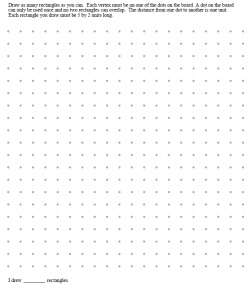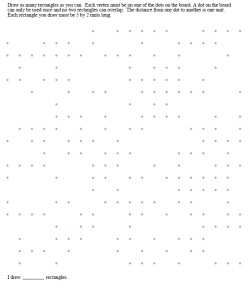edHelper.com Math Critical Thinking Geometry Drawing Shapes Challenge Lesson - Dot Game - Printable Activity Lesson Plan and Worksheets Drawing Shapes Challenge with Sizes GivenWorksheets, Lessons, and Printables

Drawing Shapes Challenge: Given One Shape and Size Requirements - Draw as many as you canDraw as many rectangles as you can - size of rectangles given Draw as many squares as you can - size of squares given Draw as many triangles as you can - size of triangles given Draw as many as you can - a shape is given (trapezoid, parallelogram, pentagon, hexagon, or octagon) Draw as many as you can - a shape is given (shape randomly picked) Four boards per page - Draw as many as you can - a shape is given (shape randomly picked)

Half of the Dots are MissingDraw as many rectangles as you can - size of rectangles given Draw as many squares as you can - size of squares given Draw as many triangles as you can - size of triangles given Draw as many as you can - a shape is given (trapezoid, parallelogram, pentagon, hexagon, or octagon) Draw as many as you can - a shape is given (shape randomly picked) Four boards per page - Draw as many as you can - a shape is given (shape randomly picked)

Drawing Shapes Challenge

Have a suggestion or would like to leave feedback?